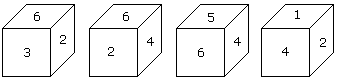# Verbal Reasoning - Dice - Discussion

### Discussion :: Dice - Dice 1 (Q.No.6)

6.

Which number is on the face opposite to 6?[A]. 4 [B]. 1 [C]. 2 [D]. 3

Explanation:

As the numbers 2, 3, 4 and 5 are adjacent to 6. Hence the number on the face opposite to 6 is 1.

 Sundar said: (Sep 8, 2012) Let me tell you an small trick to answer this kind of question in exams. Just look carefully the first dice, from that we can find that 3 and 2 are adjacent to 6 (that means option D, C cannot be the answer). Look the second dice, from that we can easily find that 4 is adjacent to 6 (Option A also cannot be the answer). Rest is option B only. (Hence the answer) !

 Binod said: (Jan 10, 2015) Check all adjacent to 6 you can easily found 1.

 Kamaljeet said: (Nov 12, 2016) Shortest answer. First, see the dice 1st and after then see dice 4th. 2 number is same place but the top change 6 places the 1. Then simply is and 6 opposite 1. And 1 opposite 6.

 Hema said: (Jun 27, 2017) Very nice explanation, thank you.

 Satya said: (Jul 27, 2017) Here we can see 3 &4 dice i.e 4 is common in both, Write the no. In anticlockwise shown below:- In 3 dice: 4,6,5 In 4 dice: 4,1,2 From above it is very clear that 6 is opposite to 1.

 Isis Rowe said: (Aug 28, 2018) What rule to follow? How to predict it?